GRE Subject Test: Math : Implicit Differentiation

Example Questions

Example Question #1 : Implicit Differentiation

Differentiate the following with respect to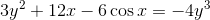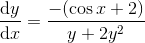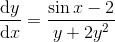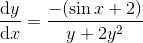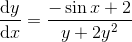Explanation:

The first step is to differentiate both sides with respect to: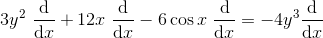Note: Those that are functions ofcan be differentiated with respect to, just remember to mulitply it by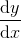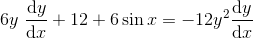Now we can solve for: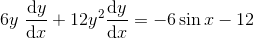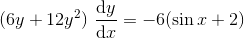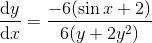Example Question #2 : Implicit Differentiation

Findfor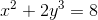.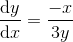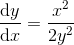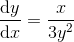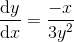Explanation:

Our first step would be to differentiate both sides with respect to: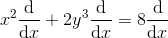The functions ofcan be differentiated with respect to, just remember to multiply by.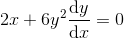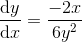Example Question #1 : Partial Differentiation

Differentiate the following to solve for.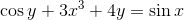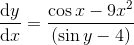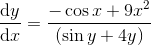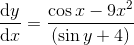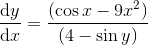Explanation:

Our first step is to differentiate both sides with respect to: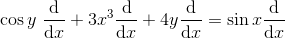The functions ofcan by differentiated with respect to, just remember to multiply them by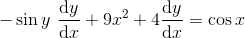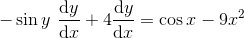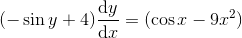Example Question #4 : Implicit Differentiation

Differentiate the following with respect to.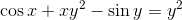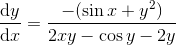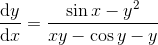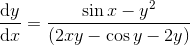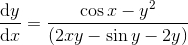Explanation:

Our first step is to differentiate both sides with respect to: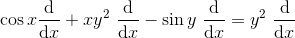Note: we can differentiate the terms that are functions ofwith respect to, just remember to multiply it by.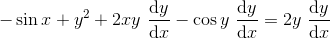Note: The product rule was applied above: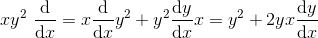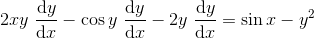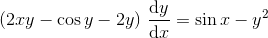All GRE Subject Test: Math Resources